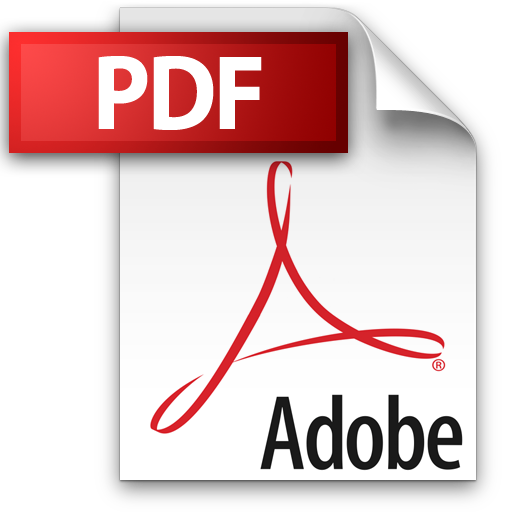``` ```FloEFD 是市面上唯一真正整合於主要 MCAD 系統的 CFD 分析軟體工具，支援輸入Pro/ENGINEER、SolidWorks、CATIA V5、Siemens NX檔案與CAD中繼檔，如Parasolid, IGES, STEP, ASIC, VDAFS, WRML, STL, IDF, DXF, DWG等格式的模型文件。此軟體一套可以快速學習及快速使用的 CFD 軟體，它完整的功能可以應用於三維氣流場及熱傳現象的分析。不同於其他傳統高階 CFD 軟體使用者需要豐富的學理基礎及網格建構技巧，其強調的是簡單操作及快速分析，所以非常適合於實際工程應用。 ``` ``` ``` `````` ``` ``` ```

### FloEFD產品特點

``` `````` ```` `

``` ```FloEFD是唯一100％直接崁入Solidworks \ Pro/E& Creo \ CATIA V5 \ NX\Solid edge 3-D CAD的熱流分析軟體，讓機構工程師可使用既有的機構模型快速地進行熱流分析，且不需要透過轉檔，減少因為轉檔造成的破面，讓熱流分析更加準確與方便。

``` `````` `````` ```

``` `````` ``` ``` `````` ```` `

``` `````` `````` ```` `

1. ``` `````` ```` `

• 自動對流體與固體區域劃分網格。

``` ```` `

• 依物理特性與外型特徵進行網格加密。

``` ```` `

• 使用者亦可利用參數控制網格的生成。

``` `````` ```

``` `````` `````` `````` ```` `

1. Modified Wall Function(改良邊界層的計算函數)

``` `````` `````` ```` `

1. ``` `````` ```` `

• 接近邊界層的網格使用partial cells技術解析。

``` ```` `

• 自動修正流動與熱傳現象的邊界層效應。

``` `````` ```

``` `````` `````` `````` ```` `

1. Laminar–Transitional–Turbulent Modelling(層流漸變到紊流的精確計算模式)

``` `````` `````` ```` `

1. ``` `````` ```` `

• 自動模擬出由層流->漸變流->紊流的現象。

``` ```` `

• 採用改良計算邊界層的函數，直接模擬層流與紊流的現象。

``` `````` ```

``` `````` `````` `````` ```` `

1. Design Variant Analysis(參數化設計分析)

``` `````` `````` ```` `

1. ``` `````` ```` `

• 採用『what-if』的設計概念，直接利用參數對模型進行優化設計。

``` ```` `

``` ```` `

• 透過參數化分析介面變更參數，比較結果。

``` `````` ```

``` `````` `````` `````` ```` `

1. EUI – Engineering User Interface(工程師使用平台)

``` `````` `````` ```` `

1. ``` `````` ```` `

``` ```` `

• Easy-to-learn：以工程師角度設計，語言相同更容易學習

``` ```` `

• 直接產生MS-Office格式的報告並輸出Excel數據與圖表。

``` `````` ```

``` `````` ```FloEFD強化應用領域的選購模組：

``` ``` ``` ```電子散熱模組(Electronic cooling module)：
``` ```

``` `````` ```` `

• 焦耳熱分析

``` ```` `

• 多孔板模組、熱管模組

``` ```` `

• 電路板模組、致冷晶片(TEC)模組

``` ```` `

• 風扇模組與風扇資料庫

``` ```` `

• 晶片資料庫

``` ```` `

• 散熱膏、散熱貼片資料庫

``` ```` `

• 更多的內建固體材料數據

``` `````` ```

``` `````` ``` ``` ```LED 散熱分析專用模組 (LED Module)： ``` ```

``` `````` ```` `

• 直接匯入T3Ster及TeraLED測試數據，

``` ```` `

• 提供2R及RC resistance network compact model，

``` ```` `

• 使用者只需要輸入電流資料，即可計算Heat Dissipation及Luminous Flux。

``` ```` `

• 輻射模組Discrete Ordinate (離散座標); 輻射模組Monte Carlo (蒙地卡羅)

``` ```` `

• 知名大廠LED資料庫

``` `````` ```

``` `````` ```通風空調模組(HVAC)： ``` ```

``` `````` ```` `

• 透光材料熱輻射效應

``` ```` `

• 熱舒適度指標分析(Predicted Mean Vote, PMV)

``` ```` `

• 不滿意的比率(Predicted of Percentage Dissatisfied, PPD)

``` ```` `

• 建材資料庫

``` `````` ```

``` `````` ```

``` ```進階應用模組(Advanced CFD)： ``` ```

``` `````` ```` `

• 超音速流

``` ```` `

• 燃燒反應

``` ```` `

• 化學反應

``` ```` `

• 蒸發與凝結二相分析

``` `````` ```

``` `````` ``` ``` ```流固耦合分析模組 (Pro/Mechanica Bridge Module & Nastran Bridge Module): ``` ```

這是專門為使用 Pro/Mechanica 以及Nastran客戶所開發的模組，可以藉由此模組將 EFD 計算出來的結構體表面溫度分布結果輸出給 Pro/Mechanica 以及Nastran格式使用，即可以計算出因為流體的溫度分布不同，包括表面溫度結果及表面壓力結果，可進行後續熱應力及結構應力應變分析。

``` `````` ``` ``` ``` ``` `````` ```

``` ```FloEFD-新版功能說明``` ```Download File

``` ```

``` ```

``` ```

``` ```

``` ```

``` ```

``` ```

``` ```

 ``` ``` ``` ``` ``` ``` ``` ``` ``` ``` ``` ``` ``` ``` ``` ```

``` ```

 ``` ``` ``` ``` ``` ``` ``` ``` ``` ``` ``` ``` ``` ``` ``` ```